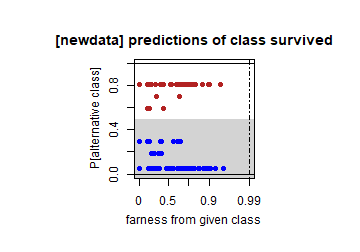# Introduction

This file illustrates the silhouette plot and class map for visualizing classification results from classification trees fit by rpart. The discussion of the results of this vignette is in section 4 of Raymaekers and Rousseeuw (2021) (for the training data), and in section A.4 of the Supplementary Material (for the test data).

``````library(rpart)
library(classmap)
``````

# Titanic training data

We first load and inspect the data.

``````data("data_titanic")

traindata = data_titanic[which(data_titanic\$dataType == "train"), -13]

dim(traindata)
``````
``````##  891  12
``````
``````colnames(traindata)
``````
``````##   "PassengerId" "Pclass"      "Name"        "Sex"         "Age"
##   "SibSp"       "Parch"       "Ticket"      "Fare"        "Cabin"
##  "Embarked"    "y"
``````
``````# SibSp: number of siblings/spouses aboard
# Parch: number of parents/children aboard

str(traindata)
``````
``````## 'data.frame':    891 obs. of  12 variables:
##  \$ PassengerId: int  1 2 3 4 5 6 7 8 9 10 ...
##  \$ Pclass     : int  3 1 3 1 3 3 1 3 3 2 ...
##  \$ Name       : chr  "Braund, Mr. Owen Harris" "Cumings, Mrs. John Bradley (Florence Briggs Thayer)" "Heikkinen, Miss. Laina" "Futrelle, Mrs. Jacques Heath (Lily May Peel)" ...
##  \$ Sex        : chr  "male" "female" "female" "female" ...
##  \$ Age        : num  22 38 26 35 35 NA 54 2 27 14 ...
##  \$ SibSp      : int  1 1 0 1 0 0 0 3 0 1 ...
##  \$ Parch      : int  0 0 0 0 0 0 0 1 2 0 ...
##  \$ Ticket     : chr  "A/5 21171" "PC 17599" "STON/O2. 3101282" "113803" ...
##  \$ Fare       : num  7.25 71.28 7.92 53.1 8.05 ...
##  \$ Cabin      : chr  "" "C85" "" "C123" ...
##  \$ Embarked   : chr  "S" "C" "S" "S" ...
##  \$ y          : Factor w/ 2 levels "casualty","survived": 1 2 2 2 1 1 1 1 2 2 ...
``````
``````table(traindata\$y)
``````
``````##
## casualty survived
##      549      342
``````

Now we want to predict the response y by CART (rpart). We set the seed as rpart is not deterministic. We also inspect the resulting classification tree.

``````set.seed(123)
rpart.out = rpart(y ~ Pclass + Sex + SibSp +
Parch + Fare + Embarked,
data=traindata, method='class', model=T)

## plot the tree:
# pdf(file = "titanic_train_tree.pdf", width=6, height=6)
rpart.plot::rpart.plot(rpart.out)
``````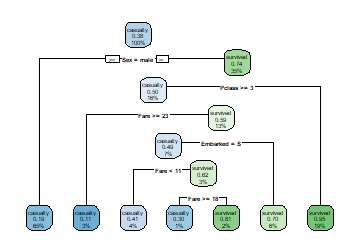``````# dev.off()
``````

We now inspect the variable importance, which will be used to calculate the variable weights in the farness computation.

``````rpart.out\$variable.importance
``````
``````##        Sex       Fare     Pclass      Parch   Embarked      SibSp
## 124.426330  43.978667  31.163132  16.994228   9.889646   8.349703
``````

Now we declare the types of the variables. This is used in the calculation of the Daisy distance, which in turn is needed for the farness computation. There are 5 nominal columns and one ordinal. The variables not listed here are interval-scaled by default.

``````mytype = list(nominal=c("Name","Sex","Ticket","Cabin","Embarked"),
ordratio=c("Pclass"))
``````

Now we prepare for visualization and inspect the elements of the output.

``````x_train = traindata[,-12]
y_train = traindata[, 12]
vcrtrain = vcr.rpart.train(x_train, y_train, rpart.out, mytype)
names(vcrtrain)
``````
``````##   "X"         "yint"      "y"         "levels"    "predint"   "pred"
##   "altint"    "altlab"    "PAC"       "figparams" "fig"       "farness"
##  "ofarness"  "trainfit"
``````
``````vcrtrain\$predint[1:10] # prediction as integer
``````
``````##   1 2 1 2 1 1 1 1 2 2
``````
``````vcrtrain\$pred[1:10]    # prediction as label
``````
``````##   "casualty" "survived" "casualty" "survived" "casualty" "casualty"
##   "casualty" "casualty" "survived" "survived"
``````
``````vcrtrain\$altint[1:10]  # alternative label as integer
``````
``````##   2 1 1 1 2 2 2 2 1 1
``````
``````vcrtrain\$altlab[1:10]  # alternative label
``````
``````##   "survived" "casualty" "casualty" "casualty" "survived" "survived"
##   "survived" "survived" "casualty" "casualty"
``````
``````# Probability of Alternative Class (PAC) of each object:
vcrtrain\$PAC[1:3]
``````
``````##  0.18890815 0.05294118 0.59459459
``````
``````#
summary(vcrtrain\$PAC)
``````
``````##    Min. 1st Qu.  Median    Mean 3rd Qu.    Max.
## 0.05294 0.18891 0.18891 0.27905 0.29630 0.94706
``````
``````# f(i,g) is the distance from case i to class g:
vcrtrain\$fig[1:3,] # for the first 3 objects:
``````
``````##           [,1]      [,2]
## [1,] 0.2621228 0.5079752
## [2,] 0.9505125 0.3728041
## [3,] 0.2303989 0.1644634
``````
``````# The farness of an object i is the f(i,g) to its own class:
vcrtrain\$farness[1:3]
``````
``````##  0.2621228 0.3728041 0.1644634
``````
``````#
summary(vcrtrain\$farness)
``````
``````##    Min. 1st Qu.  Median    Mean 3rd Qu.    Max.
##  0.0000  0.0000  0.2437  0.3286  0.6234  0.9613
``````
``````# The "overall farness" of an object is defined as the
# lowest f(i,g) it has to any class g (including its own):
summary(vcrtrain\$ofarness)
``````
``````##    Min. 1st Qu.  Median    Mean 3rd Qu.    Max.
##  0.0000  0.0000  0.1919  0.2906  0.5342  0.9578
``````
``````sum(vcrtrain\$ofarness > 0.99, na.rm = T)
``````
``````##  0
``````
``````# No farness is considered outlying in these data.

confmat.vcr(vcrtrain)
``````
``````##
## Confusion matrix:
##           predicted
## given      casualty survived
##   casualty      521       28
##   survived      130      212
##
## The accuracy is 82.27%.
``````
``````cols = c("firebrick", "blue")
``````

We first make the stacked plot, visualizing the confusion matrix:

``````stackedplot(vcrtrain, classCols = cols)
``````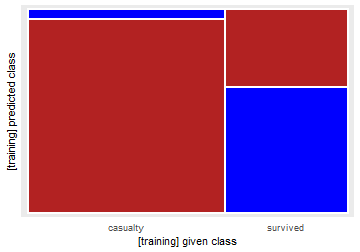Now we consider the silhouette plot

``````silplot(vcrtrain, classCols = cols)
``````
``````##  classNumber classLabel classSize classAveSi
##            1   casualty       549       0.55
##            2   survived       342       0.27
``````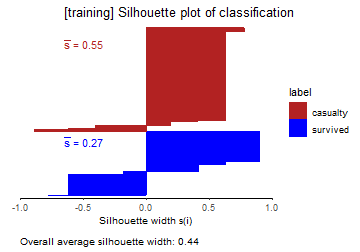``````# silplot.out <- silplot(vcrtrain, classCols = cols)
# ggsave("titanic_train_silhouettes.pdf", silplot.out,
#        width = 5, height = 5)
``````

Now we construct the quasi residual plot of PAC vs. age for males only. The age variable is not in the model, but including it did not improve the classification. We see that the very young male passengers are often misclassified.

``````hist(x_train\$Age)
``````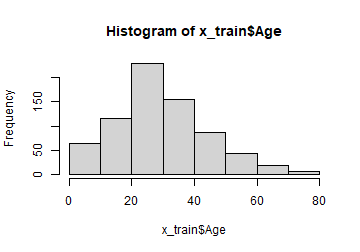``````# Quasi residual plot versus age, for males only:
xm = x_train\$Age[which(x_train\$Sex == "male")]
ym = vcrtrain\$PAC[which(x_train\$Sex == "male")]
#
# pdf("titanic_qrp_versus_age_males.pdf", width=4.8, height=4.8)
par(mar = c(3.5, 2, 2, 0))
par(pty="s") # makes the axes of the plot equally long
plot(xm, ym, type= "n", ann = FALSE, axes = FALSE)
# makes an empty plot
title(main = "quasi residual plot for males", line = 1,
cex.main = 1.2)
title(ylab = "P[alternative class]", line = 2.3,
cex.lab = 1)
title(xlab = "Age (years)", line = 2.3, cex.lab = 1)
par(new=TRUE) # overlay next plot on this one
plot(xm, ym, xlab = "", ylab = "")
lom = loess(ym ~ xm)
lines(0:82, predict(lom, 0:82), col="red", lwd = 2)
text(x=17,y=0.56,"loess curve",col="red", cex = 1)
``````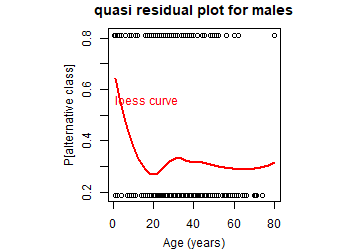``````# dev.off()

ind = which(!is.na(xm))
cor.test(xm[ind], ym[ind], method="spearman")
``````
``````## Warning in cor.test.default(xm[ind], ym[ind], method = "spearman"): Cannot
## compute exact p-value with ties
``````
``````##
##  Spearman's rank correlation rho
##
## data:  xm[ind] and ym[ind]
## S = 16706041, p-value = 0.0961
## alternative hypothesis: true rho is not equal to 0
## sample estimates:
##         rho
## -0.07828186
``````
``````# p-value is 0.0961 so not significantly different from zero.
# However, loess shows the local effect at very young age.
``````

We can do the same for females, where the effect of age is clearly much smaller:

``````xf = x_train\$Age[which(x_train\$Sex == "female")]
yf = vcrtrain\$PAC[which(x_train\$Sex == "female")]
plot(xf,yf)
lof = loess(yf ~ xf)
lines(0:82, predict(lof, 0:82), col="red")
``````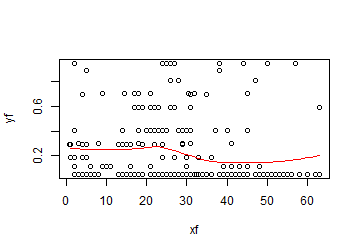Now we construct the class maps. First of the casualties.

``````classmap(vcrtrain, "casualty", classCols = cols)
``````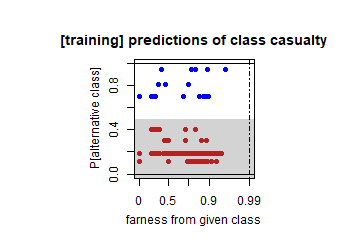``````# classmap(vcrtrain, "casualty", classCols = cols, identify = T)

# blue points top right: cases "a" and "b" in the paper
x_train[which(y_train == "casualty"), ]
``````
``````##     PassengerId Pclass                       Name    Sex Age SibSp Parch
## 178         178      1 Isham, Miss. Ann Elizabeth female  50     0     0
##       Ticket    Fare Cabin Embarked
## 178 PC 17595 28.7125   C49        C
``````
``````# Woman in 1st class, should have survived.
#
x_train[which(y_train == "casualty"), ]
``````
``````##     PassengerId Pclass                         Name    Sex Age SibSp Parch
## 298         298      1 Allison, Miss. Helen Loraine female   2     1     2
##     Ticket   Fare   Cabin Embarked
## 298 113781 151.55 C22 C26        S
``````
``````# Similar, but child.

# red point most to the right: case "c" in the paper
x_train[which(y_train == "casualty"), ]
``````
``````##     PassengerId Pclass              Name  Sex Age SibSp Parch Ticket Fare
## 439         439      1 Fortune, Mr. Mark male  64     1     4  19950  263
##           Cabin Embarked
## 439 C23 C25 C27        S
``````

Now the class map of the survivors.

``````classmap(vcrtrain, "survived", classCols = cols)
``````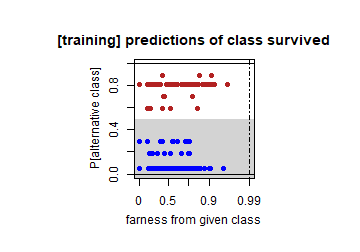``````# classmap(vcrtrain, "survived", classCols = cols, identify = T)

# red point with highest farness among highest PAC: case "d" in the paper
x_train[which(y_train == "survived")[c(14)], ]
``````
``````##    PassengerId Pclass                                                      Name
## 26          26      3 Asplund, Mrs. Carl Oscar (Selma Augusta Emilia Johansson)
##       Sex Age SibSp Parch Ticket    Fare Cabin Embarked
## 26 female  38     1     5 347077 31.3875              S
``````
``````# near-coinciding points with highest farness: cases "e" and "f" in the paper
#
x_train[which(y_train == "survived"), ]
``````
``````##     PassengerId Pclass                               Name  Sex Age SibSp Parch
## 680         680      1 Cardeza, Mr. Thomas Drake Martinez male  36     0     1
##       Ticket     Fare       Cabin Embarked
## 680 PC 17755 512.3292 B51 B53 B55        C
``````
``````x_train[which(y_train == "survived"), ]
``````
``````##     PassengerId Pclass                   Name  Sex Age SibSp Parch   Ticket
## 738         738      1 Lesurer, Mr. Gustave J male  35     0     0 PC 17755
##         Fare Cabin Embarked
## 738 512.3292  B101        C
``````
``````# man --> predicted as not survived. also paid the highest
# fare in the data and has same ticket number as passenger
# 265. Also embarked as same place. these may be related?
# according to following links they were colleagues working
# for the Cardeza family:
# https://www.encyclopedia-titanica.org/titanic-survivor/thomas-cardeza.html
# https://www.encyclopedia-titanica.org/titanic-survivor/gustave-lesueur.html

# blue point bottom right: case "g" in the paper
x_train[which(y_train == "survived"), ]
``````
``````##     PassengerId Pclass             Name    Sex Age SibSp Parch   Ticket
## 259         259      1 Ward, Miss. Anna female  35     0     0 PC 17755
##         Fare Cabin Embarked
## 259 512.3292              C
``````
``````# # woman  + first class so predicted in survivors.
# # paid highest fare in whole dataset
``````

# Titanic test data

In addition to the training data, we consider the titanic test data. First we load the data and inspect.

``````testdata = data_titanic[which(data_titanic\$dataType == "test"), -13]

dim(testdata)
``````
``````##  418  12
``````
``````x_test = testdata[, -12]
y_test = testdata[, 12]
table(y_test)
``````
``````## y_test
## casualty survived
##      260      158
``````

Now we prepare for visualization:

``````vcrtest = vcr.rpart.newdata(x_test, y_test, vcrtrain)

confmat.vcr(vcrtest)
``````
``````##
## Confusion matrix:
##           predicted
## given      casualty survived
##   casualty      230       30
##   survived       64       94
##
## The accuracy is 77.51%.
``````
``````cols = c("firebrick", "blue")
``````

Now we can visualize, starting with the stacked plot:

``````stackedplot(vcrtest, classCols=cols)
``````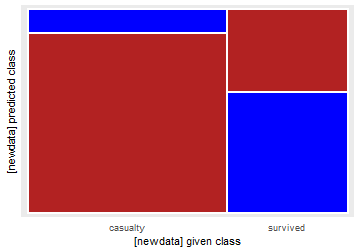Now we construct the silhouette plot:

``````silplot(vcrtest, classCols = cols)
``````
``````##  classNumber classLabel classSize classAveSi
##            1   casualty       260       0.47
##            2   survived       158       0.25
``````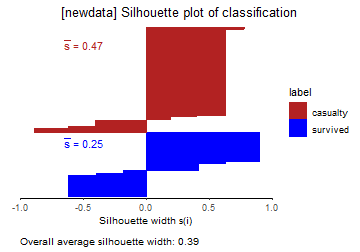Finally, we make the class maps. First of the casualties, in which the misclassifications are all female (since all males are predicted as casualty by this model).

``````classmap(vcrtest, "casualty", classCols = cols)
``````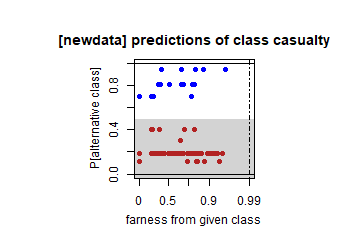Now the class map of survivors:

``````classmap(vcrtest, "survived", classCols = cols)
``````